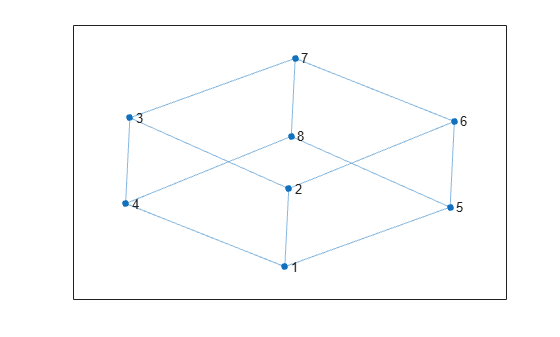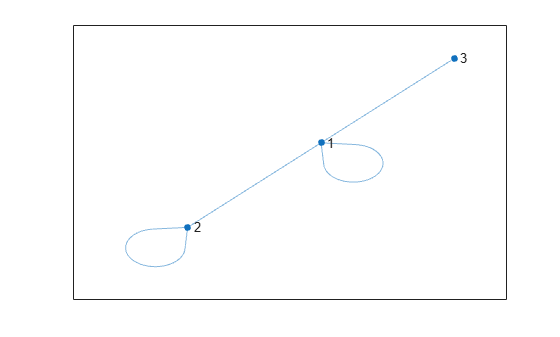# rmedge

Remove edge from graph

## Syntax

``H = rmedge(G,s,t)``
``H = rmedge(G,idx)``

## Description

example

````H = rmedge(G,s,t)` removes the edges specified by the node pairs `s` and `t` from graph `G`. If there are multiple edges specified by `s` and `t`, then they are all removed.```

example

````H = rmedge(G,idx)` specifies which edges to remove with edge indices `idx`. The edge indices are row numbers in the `G.Edges` table.```

## Examples

collapse all

Create and plot a graph.

```s = [1 1 1 2 2 3 3 4 5 5 6 7]; t = [2 4 5 3 6 4 7 8 6 8 7 8]; G = graph(s,t); plot(G)```Remove several edges from the graph and plot the result.

```G = rmedge(G,[1 2 3 4],[5 6 7 8]); plot(G)```Create a graph and view the edge list.

```s = {'BOS' 'NYC' 'NYC' 'NYC' 'LAX'}; t = {'NYC' 'LAX' 'DEN' 'LAS' 'DCA'}; G = digraph(s,t); G.Edges```
```ans=5×1 table EndNodes __________________ {'BOS'} {'NYC'} {'NYC'} {'LAX'} {'NYC'} {'DEN'} {'NYC'} {'LAS'} {'LAX'} {'DCA'} ```

Remove the edge between nodes `'NYC'` and `'DEN'` using the edge index.

```G = rmedge(G,3); G.Edges```
```ans=4×1 table EndNodes __________________ {'BOS'} {'NYC'} {'NYC'} {'LAX'} {'NYC'} {'LAS'} {'LAX'} {'DCA'} ```

This example shows how to remove all of the self-loops from a graph. Self-loops are edges that connect a node to itself.

Create a graph that has two self-loops.

```G = graph([1 1 1 2],[1 2 3 2]); plot(G)```Use `rmedge` to remove all self-loops from the graph. Even though `G` has only two self-loops, this technique removes all self-loops from any directed or undirected graph.

```G = rmedge(G, 1:numnodes(G), 1:numnodes(G)); plot(G)```## Input Arguments

collapse all

Input graph, specified as either a `graph` or `digraph` object. Use `graph` to create an undirected graph or `digraph` to create a directed graph.

Example: `G = graph(1,2)`

Example: `G = digraph([1 2],[2 3])`

Node pairs, specified as separate arguments of node indices or node names. Similarly located elements in `s` and `t` specify the source and target nodes for edges in the graph.

This table shows the different ways to refer to one or more nodes either by their numeric node indices or by their node names.

FormSingle NodeMultiple Nodes
Node index

Scalar

Example: `1`

Vector

Example: `[1 2 3]`

Node name

Character vector

Example: `'A'`

Cell array of character vectors

Example: `{'A' 'B' 'C'}`

String scalar

Example: `"A"`

String array

Example: `["A" "B" "C"]`

Example: `G = rmedge(G,1,2)` removes the edge between node 1 and node 2 from graph `G`.

Example: `G = rmedge(G,{'a' 'b'},{'d' 'c'})` removes two edges from graph `G`, the first of which is between node `'a'` and node `'d'`.

Edge indices, specified as a scalar or vector. The edge indices are nonnegative integers that are row numbers in the `G.Edges` table.

Example: `G = rmedge(G,[1 3 5])` removes the first, third, and fifth edges (rows) from `G.Edges`.

## Output Arguments

collapse all

Output graph, returned as a `graph` or `digraph` object.

## Version History

Introduced in R2015b